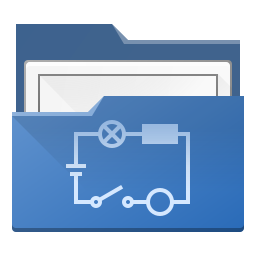QElectroTech  0.8-dev
QetGraphicsTableFactory Class Reference

The QetGraphicsTableFactory class. More...

#include <qetgraphicstablefactory.h>

Collaboration diagram for QetGraphicsTableFactory: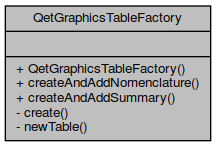Public Member Functions

QetGraphicsTableFactory ()

Static Public Member Functions

QetGraphicsTableFactory::createAndAddNomenclature Open a dialog for ask user the config of the table, create a nomenclature table and add it to diagram. More...

QetGraphicsTableFactory::createAndAddSummary Open a dialog for ask user the config of the table, create a summary table and add it to diagram. More...

Static Private Member Functions

static void create (Diagram *diagram, AddTableDialog *dialog)

static QetGraphicsTableItemnewTable (Diagram *diagram, AddTableDialog *dialog, QetGraphicsTableItem *previous_table=nullptr)
QetGraphicsTableFactory::newTable Create a new table . More...

Detailed Description

The QetGraphicsTableFactory class.

◆ QetGraphicsTableFactory()

 QetGraphicsTableFactory::QetGraphicsTableFactory ( )

◆ create()

 void QetGraphicsTableFactory::create ( Diagram * diagram, AddTableDialog * dialog )
staticprivate
Here is the call graph for this function: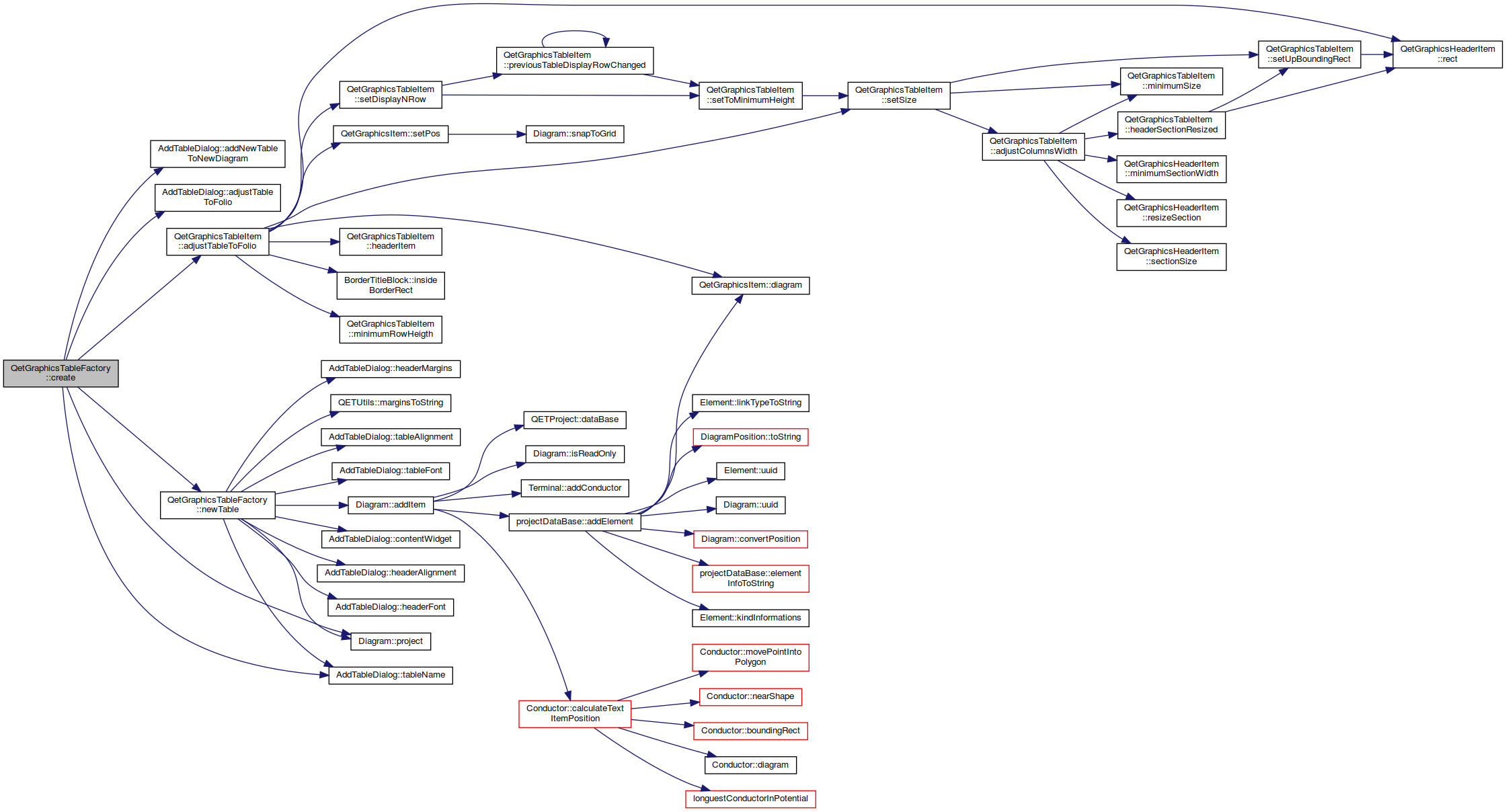Here is the caller graph for this function: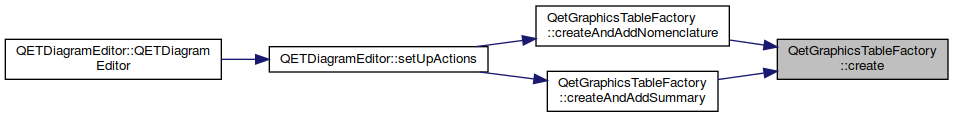void QetGraphicsTableFactory::createAndAddNomenclature ( Diagram * diagram )
static

QetGraphicsTableFactory::createAndAddNomenclature Open a dialog for ask user the config of the table, create a nomenclature table and add it to diagram.

Parameters
 diagram
Here is the call graph for this function: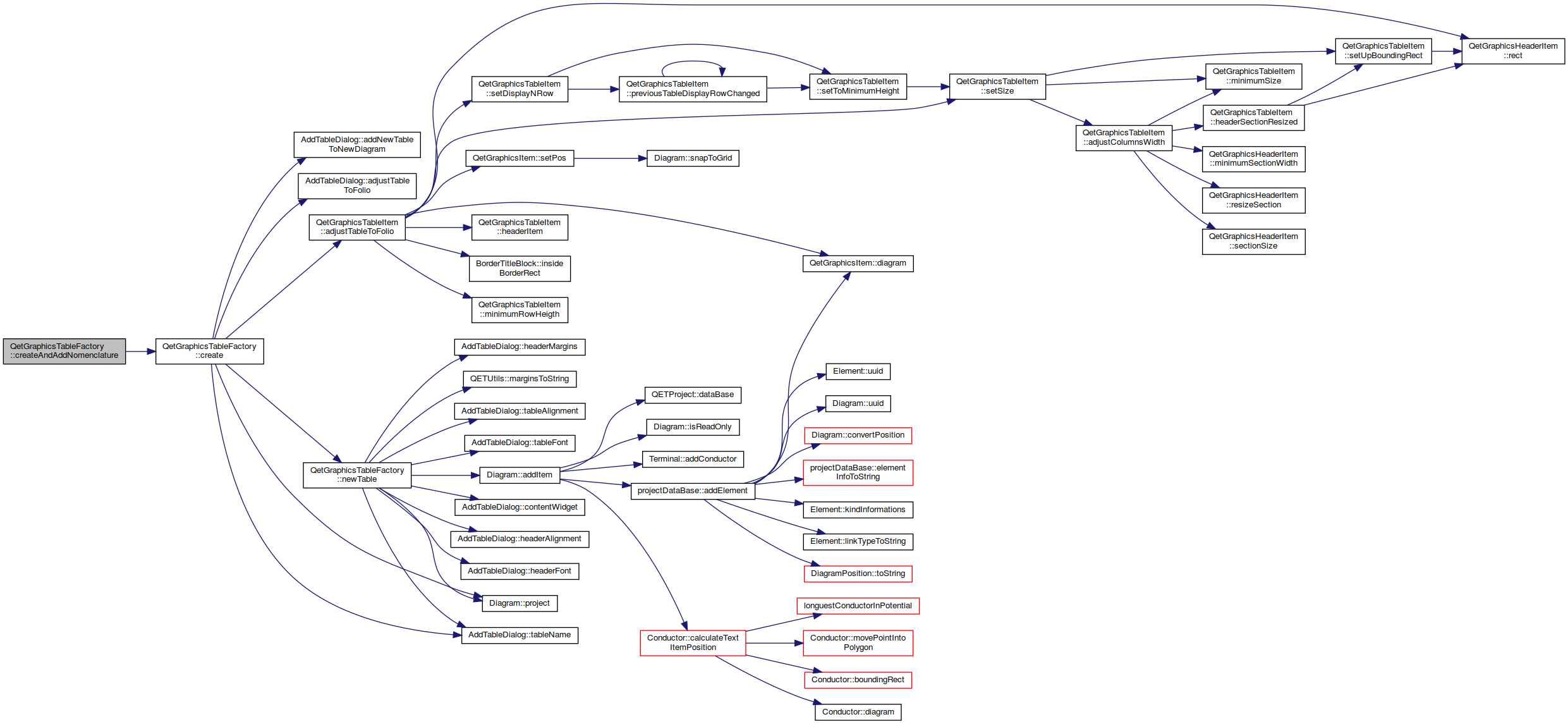Here is the caller graph for this function: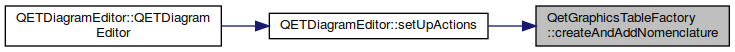void QetGraphicsTableFactory::createAndAddSummary ( Diagram * diagram )
static

QetGraphicsTableFactory::createAndAddSummary Open a dialog for ask user the config of the table, create a summary table and add it to diagram.

Parameters
 diagram
Here is the call graph for this function: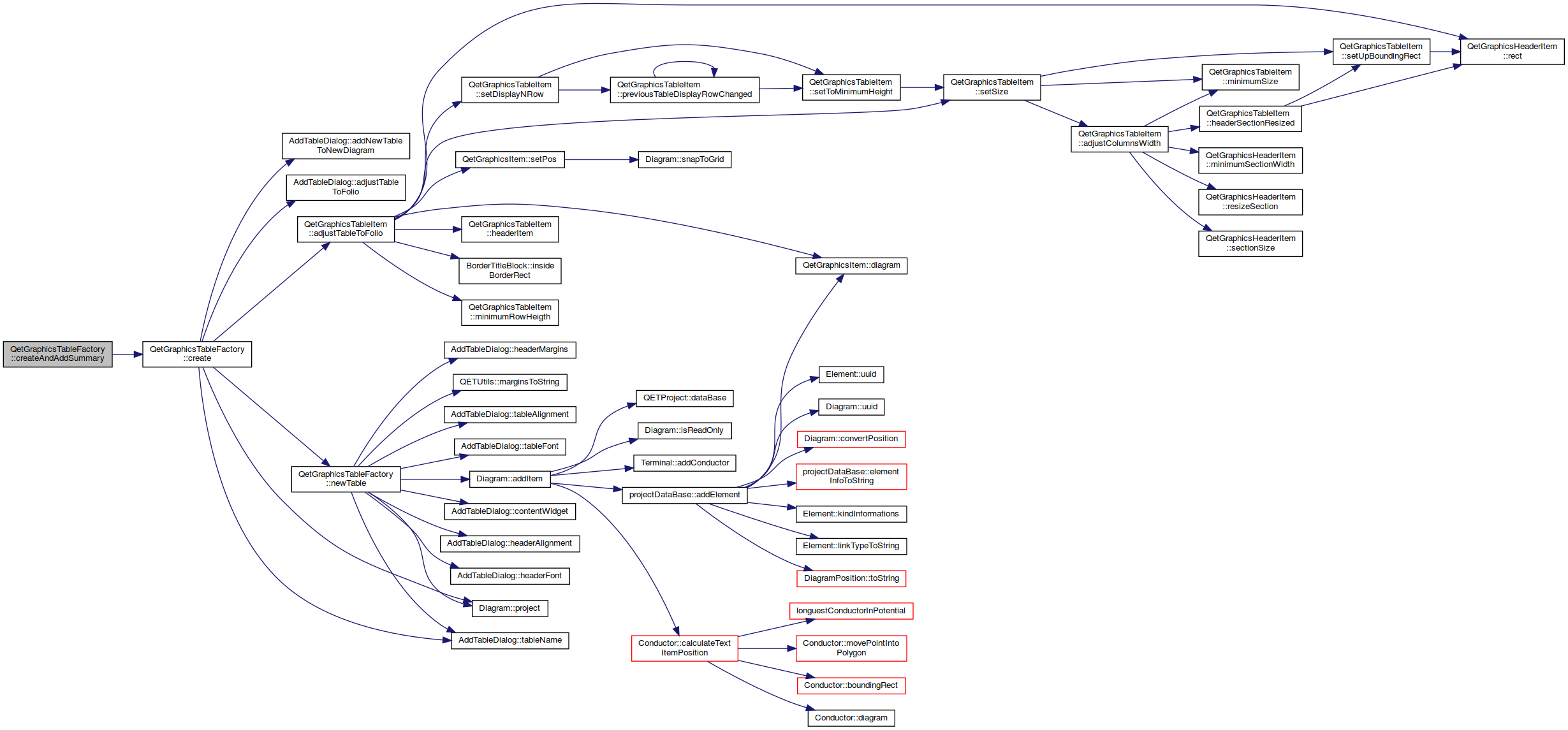Here is the caller graph for this function: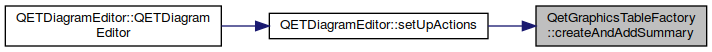◆ newTable()

 QetGraphicsTableItem * QetGraphicsTableFactory::newTable ( Diagram * diagram, AddTableDialog * dialog, QetGraphicsTableItem * previous_table = nullptr )
staticprivate

QetGraphicsTableFactory::newTable Create a new table .

Parameters
 diagram : Diagram where we must add the new table. dialog : dialog conf, it's used to setup the model. previous_table : If you know that the new table will have a previous table and you already now the previous table, set it now they will improve time needed for creating the new table by avoiding to create a new model.
Returns
the new table
Here is the call graph for this function: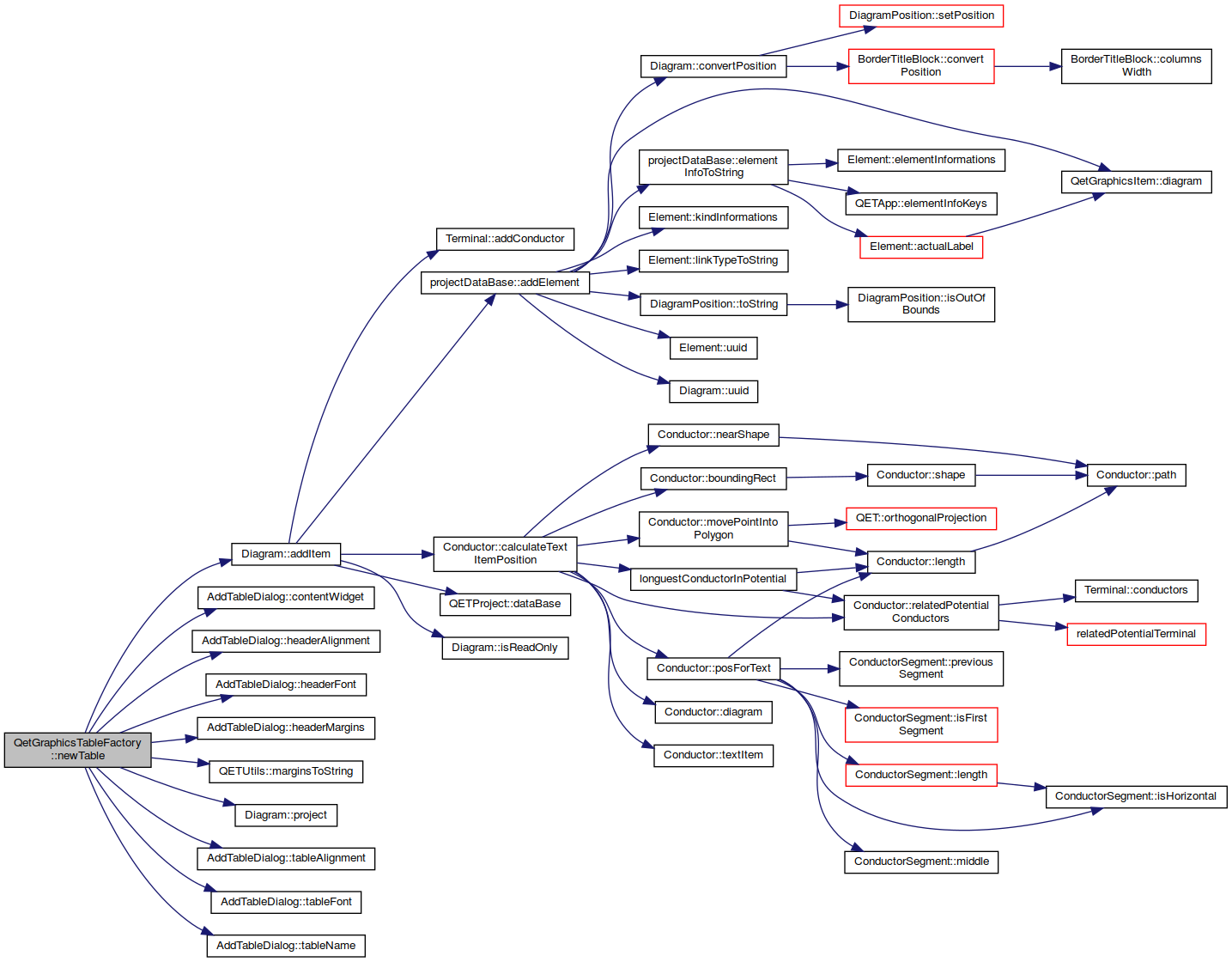Here is the caller graph for this function: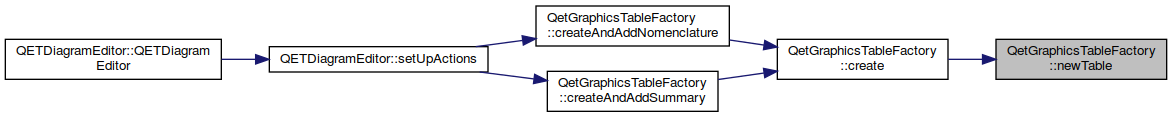The documentation for this class was generated from the following files: